Home > CCA2 > Chapter 11 > Lesson 11.1.1 > Problem11-10

11-10.
1. Natural logs and exponential functions with base e are often used in formulas. But many problems can be solved equally well using either a base-10 logarithm or a base-e logarithm. Solve each of the following problems, first using the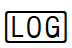key (base 10) and then using the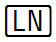key (base e) on your calculator. Homework Help ✎

1. 10,000(1.08)x = 20,000

2. 30,000(0.8)x = 15,000

3. Interpret the answer for part (a) if the equation represents an amount of money invested at 8% annual interest.

4. Interpret the answer for part (b) if the equation represents the price paid for a car that depreciates at 20% per year.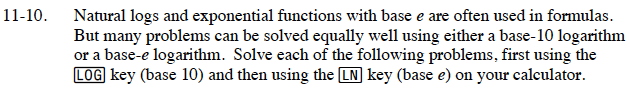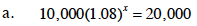log(1.08x) = log2

x · log(1.08) = log2

9.00646832

You should get the same answer using natural logs.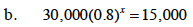See part (a).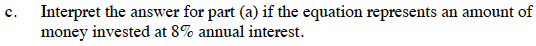What does x represent in this equation?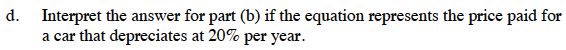What does x represent in this equation?